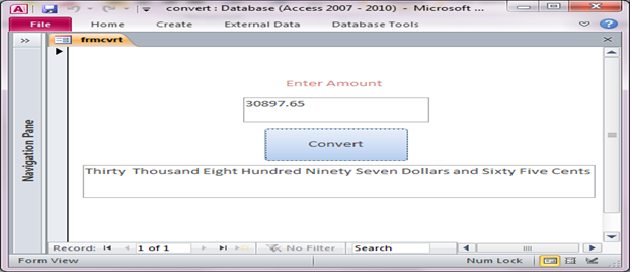# Convert currency into words in Microsoft Access using VBA

Many times we need the amount in figures to be converted into words. Converters usually display a value that is useful in accounting and invoicing and preparing financial plans and reports. This is a typical requirement for writing checks or any other financial reports.

In this article we are convert currency which is given in number into words, we can use it into report and invoice coding where we can change easily number/currency into words. For implementation that code firstly we have to create the Form with following design as shown in Fig 1.1.Fig:-1.1

You can see here after code implement in figure 1.2.Fig:-1.2

VBA CODE :

Option Compare Database
Private Sub btn1_Click()
Me!txt2.Value = wsiSpellNumber(Me!txt1.Value)
End Sub
Public Function wsiSpellNumber(ByVal MyNumber)
Dim Dollars, Cents, Temp
Dim DecimalPlace, Count
ReDim Place(9) As String
Place(2) = " Thousand "
Place(3) = " Million "
Place(4) = " Billion "
Place(5) = " Trillion "
' String representation of amount.
MyNumber = Trim(Str(MyNumber))
' Position of decimal place 0 if none.
DecimalPlace = InStr(MyNumber, ".")
' Convert cents and set MyNumber to dollar amount.
If DecimalPlace > 0 Then
Cents = GetTens(Left(Mid(MyNumber, DecimalPlace + 1) & _ "00", 2))
MyNumber = Trim(Left(MyNumber, DecimalPlace - 1))
End If
Count = 1
Do While MyNumber <> ""
Temp = GetHundreds(Right(MyNumber, 3))
If Temp <> "" Then Dollars = Temp & Place(Count) & Dollars
If Len(MyNumber) > 3 Then
MyNumber = Left(MyNumber, Len(MyNumber) - 3)
Else
MyNumber = ""
End If
Count = Count + 1
Loop
Select Case Dollars
Case ""
Dollars = "No Dollars"
Case "One"
Dollars = "One Dollar"
Case Else
Dollars = Dollars & " Dollars"
End Select
Select Case Cents
Case ""
Cents = " and No Cents"
Case "One"
Cents = " and One Cent"
Case Else
Cents = " and " & Cents & " Cents"
End Select
wsiSpellNumber = Dollars & Cents
End Function
' Converts a number from 100-999 into text
Function GetHundreds(ByVal MyNumber)
Dim result As String
If Val(MyNumber) = 0 Then Exit Function
MyNumber = Right("000" & MyNumber, 3)
' Convert the hundreds place.
If Mid(MyNumber, 1, 1) <> "0" Then
result = GetDigit(Mid(MyNumber, 1, 1)) & " Hundred "
End If
' Convert the tens and ones place.
If Mid(MyNumber, 2, 1) <> "0" Then
result = result & GetTens(Mid(MyNumber, 2))
Else
result = result & GetDigit(Mid(MyNumber, 3))
End If
GetHundreds = result
End Function
' Converts a number from 10 to 99 into text.
Function GetTens(TensText)
Dim result As String
result = "" ' Null out the temporary function value.
If Val(Left(TensText, 1)) = 1 Then ' If value between 10-19...
Select Case Val(TensText)
Case 10: result = "Ten"
Case 11: result = "Eleven"
Case 12: result = "Twelve"
Case 13: result = "Thirteen"
Case 14: result = "Fourteen"
Case 15: result = "Fifteen"
Case 16: result = "Sixteen"
Case 17: result = "Seventeen"
Case 18: result = "Eighteen"
Case 19: result = "Nineteen"
Case Else
End Select
Else ' If value between 20-99...
Select Case Val(Left(TensText, 1))
Case 2: result = "Twenty "
Case 3: result = "Thirty "
Case 4: result = "Forty "
Case 5: result = "Fifty "
Case 6: result = "Sixty "
Case 7: result = "Seventy "
Case 8: result = "Eighty "
Case 9: result = "Ninety "
Case Else
End Select
result = result & GetDigit _ (Right(TensText, 1)) ' Retrieve ones place.
End If
GetTens = result
End Function
' Converts a number from 1 to 9 into text.
Function GetDigit(Digit)
Select Case Val(Digit)
Case 1: GetDigit = "One"
Case 2: GetDigit = "Two"
Case 3: GetDigit = "Three"
Case 4: GetDigit = "Four"
Case 5: GetDigit = "Five"
Case 6: GetDigit = "Six"
Case 7: GetDigit = "Seven"
Case 8: GetDigit = "Eight"
Case 9: GetDigit = "Nine"
Case Else: GetDigit = ""
End Select
End Function

DISCLAIMER

It is advised that the information provided in the article should not be used for any kind formal or production programming purposes as content of the article may not be complete or well tested. ERP Makers will not be responsible for any kind of damage (monetary, time, personal or any other type) which may take place because of the usage of the content in the article.